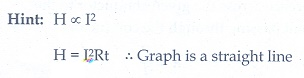# Unit II: Current Electricity - Online Test

Q1. What is the current out of the battery?Explaination / Solution: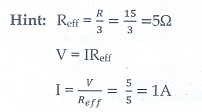Q2. The temperature coefficient of resistance of a wire is 0.00125 per °C. At 300 K, its resistance is 1 Ω. The resistance of the wire will be 2 Ω at
Explaination / Solution: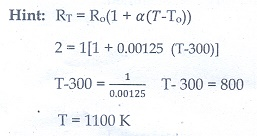Q3. The internal resistance of a 2.1 V cell which gives a current of 0.2 A through a resistance of 10 Ω is
Explaination / Solution: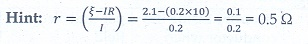Q4. A piece of copper and another of germanium are cooled from room temperature to 80 K. The resistance of
Explaination / Solution: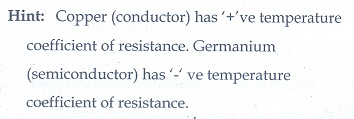Q5. In Joule’s heating law, when I and t are constant, if the H is taken along the y axis and I2 along the x axis, the graph is
Explaination / Solution: